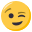# C++ Primer 3.3.3

• Hey, this is my program:

``````std::vector<int> test(5);
int a, b, c, d, s;
std::cin >> a >> b >> c >> d;
test.push_back(a);
test.push_back(b);
test.push_back(c);
test.push_back(d);
s = test + test ;
std::cout << s << std::endl;
s = test + test ;
std::cout << s << std::endl;
s = test + test ;
std::cout << s << std::endl;
s = test + test ;
std::cout << s << std::endl;
``````

it shall print the sum of the pairs and the sum of the first and last number in 'test'. I did write and read stuff this way already with strings, but now when i use ints, it doesn't work ...

if i input '1 2 3 4' the output is '0 0 1 1'
if i input '1 1 1 1' its the same.

thanks.

Benno

• hihohohi schrieb:

Hey, this is my program:

``````std::vector<int> test(5);
int a, b, c, d, s;
std::cin >> a >> b >> c >> d;
test.push_back(a);
test.push_back(b);
test.push_back(c);
test.push_back(d);
s = test + test ;
std::cout << s << std::endl;
s = test + test ;
std::cout << s << std::endl;
s = test + test ;
std::cout << s << std::endl;
s = test + test ;
std::cout << s << std::endl;
``````

it shall print the sum of the pairs and the sum of the first and last number in 'test'. I did write and read stuff this way already with strings, but now when i use ints, it doesn't work ...

if i input '1 2 3 4' the output is '0 0 1 1'
if i input '1 1 1 1' its the same.

thanks.

Benno

sum of pairs:

``````s = test + test ;
std::cout << s << std::endl;
s = test + test ;
std::cout << s << std::endl;
s = test + test ;
std::cout << s << std::endl;
``````

so you dont need s.

``````std::vector<int> test;//not initiallly fillng with 5 zeros
``````

• oh ya the pushback function adds to the endthanks man, works now.

``````std::vector<int> test;
int a, b, c, d, e, s;
std::cin >> a >> b >> c >> d;
test.push_back(a);
test.push_back(b);
test.push_back(c);
test.push_back(d);
test.push_back(e);
s = test + test ;
std::cout << s << std::endl;
s = test + test ;
std::cout << s << std::endl;
s = test + test ;
std::cout << s << std::endl;
``````

• If you want to optimize try using std::vector::reserve. It allocates memory for n elements, but the size stays at the old value (in this case 0), so push_back will result in the way you need it.

But in this special case it might be no optimization due to too less values. Long story short, you wont need it• Skym0sh0 schrieb:

If you want to optimize try using std::vector::reserve. It allocates memory for n elements, but the size stays at the old value (in this case 0), so push_back will result in the way you need it.

But in this special case it might be no optimization due to too less values. Long story short, you wont need iti will keep that in mind for later tasksthanks